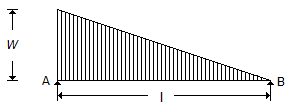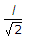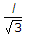# Mechanical Engineering - Strength of Materials

Exercise : Strength of Materials - Section 1
31.
The bending stress in a beam is __________ section modulus.
directly proportional to
inversely proportional to
Explanation:
No answer description is available. Let's discuss.

32.
When shear force at a point is zero, then bending moment is __________ at that point.
zero
minimum
maximum
infinity
Explanation:
No answer description is available. Let's discuss.

33.
The maximum bending moment for the beam shown in the below figure, lies at a distance of __________ from the end B.l/2
l/3Explanation:
No answer description is available. Let's discuss.

34.
When a bar is cooled to - 5°C, it will develop
no stress
shear stress
tensile stress
compressive stress